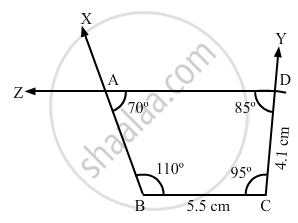Share

# Construct a Quadrilateral Abcd When Bc = 5.5 Cm, Cd = 4.1 Cm, ∠A = 70°, ∠B = 110° and ∠D = 85°. - Mathematics

Course
ConceptConstructing a Quadrilateral When Two Adjacent Sides and Three Angles Are Known

#### Question

Construct a quadrilateral ABCD when BC = 5.5 cm, CD = 4.1 cm, ∠A = 70°, ∠B = 110° and ∠D = 85°.

#### Solution

We know that the sum of all the angles in a quadrilateral is 360 .

$i . e . , \angle A + \angle B + \angle C + \angle D = 360^\circ$

$\Rightarrow \angle C = 95^\circ$

Steps of construction:

Step I: Draw BC = 5 . 5 cm .

$\text { Step II: Construct } \angle XBC = 110^\circ\text { at A and }\angle BCY = 95^\circ .$

Step III: With C as the centre and radius 4 . 1 cm, cut off CD = 4 . 1 cm .

$\text { Step IV: At D, draw } \angle CDZ = 85^\circ \text {P such that it meets BY at A } .$